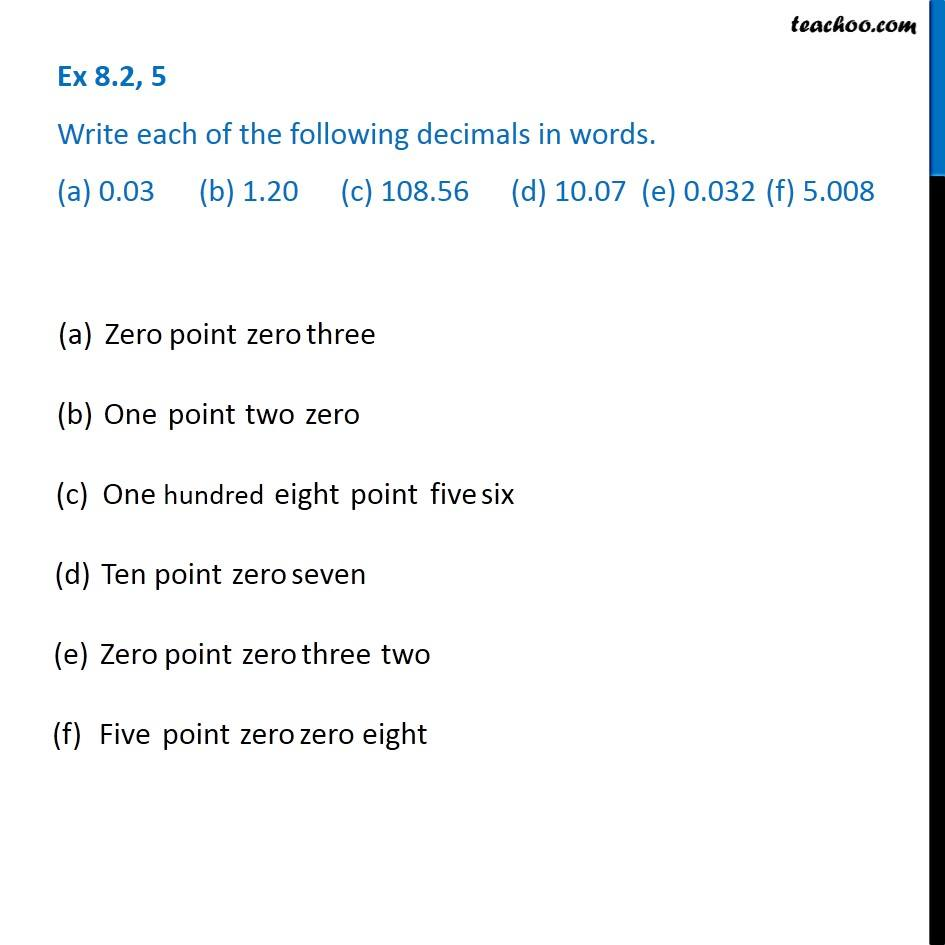Writing Fractions as Decimals worksheet

Chapter 8 Class 6 Decimals
Serial order wiseLearn in your speed, with individual attention - Teachoo Maths 1-on-1 Class

### Transcript

Question 5 - Chapter 8 Class 6 Write each of the following decimals in words. (a) 0.03 (b) 1.20 (c) 108.56 (d) 10.07 (e) 0.032 (f) 5.008 (a) Zero point zero three (b) One point two zero (c) One hundred eight point five six (d) Ten point zero seven (e) Zero point zero three two (f) Five point zero zero eight